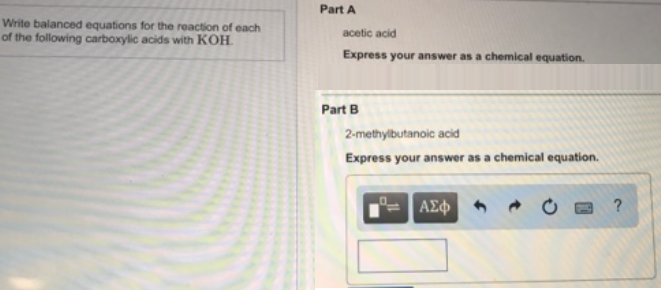# Problem: Write balanced equations for the reaction of each of the following carboxylic acids with KOH. Part A acetic acid Express your answer as a chemical equation. Part B 2-methylbutanoic acid Express your answer as a chemical equation.

🤓 Based on our data, we think this question is relevant for Professor Urhoghide's class at MDC.

###### FREE Expert Solution###### Problem Details

Write balanced equations for the reaction of each of the following carboxylic acids with KOH.

Part A

acetic acid

Part B

2-methylbutanoic acid